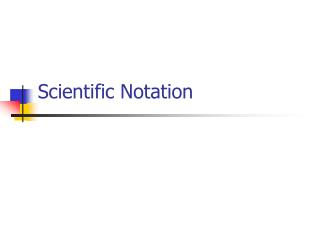DownloadDownload PresentationScientific Notation

# Scientific Notation

Download Presentation## Scientific Notation

- - - - - - - - - - - - - - - - - - - - - - - - - - - E N D - - - - - - - - - - - - - - - - - - - - - - - - - - -
##### Presentation Transcript

1. Scientific Notation

2. Scientific Notation • A number is in scientific notation when • There is a number (I’ll call “n”) that is bigger than or equal to one, but less than ten. • This number “n” is multiplied by a power of ten. • A power of ten is 10 to the something power. • So it will look something like this: • N x 10 -3

3. Which are in scientific notation? 3.5 x 103 62.6 x 10-2 0.86 x 108 3.82 x 100-6 4.6 x 100 Yes No No No Yes Examples

4. Writing Numbers in Scientific Notation • Move the decimal so that the new number is greater than or equal to one, but less than ten (so that there is one nonzero number to the left of the decimal). • Count the number of places you moved the decimal (This will be the exponent of 10) • If the number started out bigger than one, the exponent will be positive. • If the number started out less than one, the exponent will be negative.

5. Write in scientific notation 13,030,000 0.000 092 0.003 5 4,825,000,000 675 million 283 hundred thousandths 1.303 x 107 9.2 x 10-5 3.5 x 10-3 4.825 x 109 6.75 x 108 2.83 x 10-3 Examples

6. To change to a standard number: • Look at the exponent on the 10. • If it is positive, MOVE the decimal that many places to the right. • If it is negative, MOVE the decimal that many places to the left.

7. Write as a standard number: 3.84 x 106 6.2 x 10-5 5 x 10-3 1.2345 x 1010 7.91 x 100 5 x (7 x 106) 3,840,000 0.000 062 0.005 12,345,000,000 7.91 35,000,000 Examples

8. Powers of 10 • When the exponent is positive, it is telling you how many zeroes you will have. • When the exponent is negative, it is telling you how many places you will have in the number.

9. 106 10-3 107 10-5 0.00001 10,000 100,000,000,000 0.01 1,000,000 0.001 10,000,000 0.00001 10-5 104 1011 10-2 Examples